# Comparing Quantities

## Quick Revision of previous class Maths concepts

### Unitary Method

Unitary method is on the most useful method to solve ratio, proportion and   percentage problems. In this we first find value of one unit and then find the value of required number of units.
So in Short Unitary method comprises two following steps:

Step 1 = Find the value of one unit.

Step 2 = Then find the value of required number of units.
Example
If 9 kg Mangoes costs Rs.450, then how much 8 Kg Mangoes will costs?
Solution
By unitary Method
9 kg Mangoes  = Rs.450
1 kg Mangoes = Rs.450/ 9 ( unit price)
8 kg Mangoes  = Rs. (450/ 9)*8 = Rs.400

### Percentages

Percentages are ways to compare quantities. They are numerators of fractions with denominator 100 or   it basically means per 100 value
Per cent is derived from Latin word ‘per centum’ meaning ‘per hundred’
It is denoted by % symbol
1% means 1/100= .01
We can use either unitary method or we need to convert the fraction to an equivalent fraction with denominator 100
Example
4 out of 10 shirts are Red. What % of shirts are red?
Solution
By Unitary method
Out of 10 shirt  ,the number of red shirts are 4
Hence, out of 100, the number of red shirts are
= (4/10)×100 = 40
So 40%
By Fraction method
The ratio  is 4/10
= (4/10) × (10/10)
=40/100
So 40%

## Finding Discounts

Discount is a reduction given on the Marked Price (MP) of the article.
This is generally given to attract customers to buy goods or to promote sales of the goods. You can find
the discount by subtracting its sale price from its marked price.
So, Discount = Marked price – Sale price
Example
In Big Bazar an item of  Rs 800 is sold for Rs 640. What is the discount and discount %?
Solution:
Discount = Marked Price – Sale Price
= Rs 800 – Rs 640
= Rs 160
Since discount is on marked price, we will have to use marked price as the base.
By unitary method
On marked price of Rs 800, the discount is Rs 160
On MP of Rs 100, how much will the discount be?
Discount = (160/800)X100=20%
By Fraction method
160/800  = (4/20) X (5/5)
= 20/100 =20%

## Profit and Loss

Cost Price :  It is the actual price of the item
Overhead charges/expenses :These additional expenses are made while buying or before selling it. These expenses have to be included in the cost price
So Cost Price:  Actual CP  + overhead charges
Selling Price: It is price at which the item is sold  to the customer
If S.P > C.P,  We make some money from selling the item. This is called Profit
Profit = SP – CP
Profit % = (P/CP) X 100
If S.P < C.P, We loose some money from selling the item. This is called Loss
Loss = C.P – S.P
Loss % =( L/C.P) X 100
Example
A shopkeeper purchased 200 Maggi for Rs 10 each. He  sold them at Rs 12 each. Find the ain or loss %.
Solution:
Cost price of 200 Maggi = RS 200 × 10 = ` 2000
The SP of 200 Maggi  = Rs 200 × 12 = Rs 2400
He obviously made a profit (as SP > CP).
Profit = Rs 2400 – Rs 2000 = Rs 400
On Rs 2000, the profit is Rs 400
On Rs 100, the profit would be
=(400/2000)X100= 20%
Watch this tutorial for more explanation About Profit and Loss

## Sales Tax and VAT

Sales Tax(ST)
This is the amount   charged by the government on the sale of an item.
It is collected by the shopkeeper from the customer and given to the government. This is, therefore, always on the selling price of an item and is added to the value of the bill.
This is the again the amount   charged by the government on the sale of an item. It is collected by the shopkeeper from the customer and given to the government. This is, therefore, always on the selling price of an item and is added to the value of the bill.
Earlier You must have seen Sales tax on the bill, now a days , you will mostly see Value Added Tax
Calculation
If the tax is x%, then Total price after including tax would be
Final Price= Cost of item + (x/cost of item) X100
Watch this tutorial for more explanation About Sales Tax and VAT including example

## Simple Interest and Compound Interest

Interest
Interest is the extra money paid by institutions like banks or post offices on money deposited (kept) with them. Interest is also paid by people when they borrow money
Simple Interest
Principal (P): The original sum of money loaned/deposited. Also known as capital.
Time (T): The duration for which the money is borrowed/deposited.
Rate of Interest (R): The percent of interest that you pay for money borrowed, or earn for money deposited
Simple interest is calculated as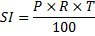Total amount at the end of time period
A=  P + SI
Compound Interest
Principal (P): The original sum of money loaned/deposited.
Time (n): The duration for which the money is borrowed/deposited.
Rate of Interest (R): The percent of interest that you pay for money borrowed, or earn for money deposited
Compound interest is the interest calculated on the previous year’s amount (A = P + I).
So Ist Year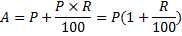IInd year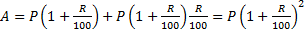So N year will be given by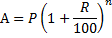The above formula is the interest compounded annually
Compound Interest Formula if the interest is compound half yearly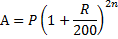Here R/2 is the half yearly rate
2n is the number of half year
Similarly, for interest compounded quarterly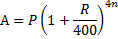Watch this tutorial for more explanation About Simple interest and Compound interest

Example
a) Find Compound interest for  Rs 12600 for 2 years at 10% per annum compounded annually.
b) Find the interest if it is not compound annually
c) Compare them
Solution:
a)where Principal (P) = Rs 12600, Rate (R) = 10,
Number of years (n) = 2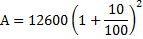=15246
CI = A – P = Rs 15246 – Rs 12600 =Rs 2646

b)
If it is not compounded annually ie. Simple interest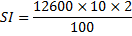SI= Rs2520
c) It is clear that
CI > SI

Reference Books for class 8 Math

Given below are the links of some of the reference books for class 8 Math.

1. Mathematics Foundation Course for JEE/Olympiad : Class 8 This book can take students maths skills further. Only buy if child is interested in Olympiad/JEE foundation courses.
2. Mathematics for Class 8 by R S Aggarwal Detailed Mathematics book to clear basics and concepts. I would say it is a must have book for class 8 student.
3. Pearson Foundation Series (IIT -JEE / NEET) Physics, Chemistry, Maths & Biology for Class 8 (Main Books) | PCMB Combo : These set of books could help your child if he aims to get extra knowledge of science and maths. These would be helpful if child wants to prepare for competitive exams like JEE/NEET. Only buy if you can provide help to the child while studying.
4. Reasoning Olympiad Workbook - Class 8 :- Reasoning helps sharpen the mind of child. I would recommend students practicing reasoning even though they are not appearing for Olympiad.

You can use above books for extra knowledge and practicing different questions.

Note to our visitors :-

Thanks for visiting our website. From feedback of our visitors we came to know that sometimes you are not able to see the answers given under "Answers" tab below questions. This might happen sometimes as we use javascript there. So you can view answers where they are available by reloding the page and letting it reload properly by waiting few more seconds before clicking the button.
We really do hope that this resolve the issue. If you still hare facing problems then feel free to contact us using feedback button or contact us directly by sending is an email at [email protected]
We are aware that our users want answers to all the questions in the website. Since ours is more or less a one man army we are working towards providing answers to questions available at our website.